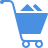Cart (0)

The standardized values are computed by subtracting the mean from each value and then dividing it by the standard deviation. (It is the same as normalized values.)

Previous Entry
Next Entry